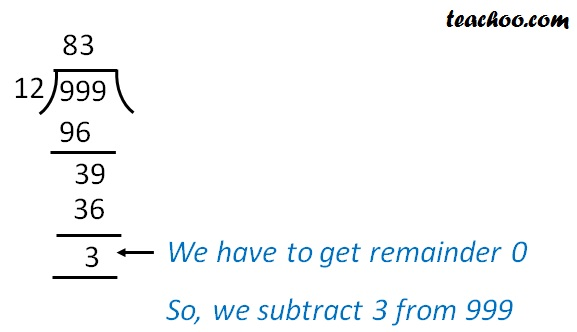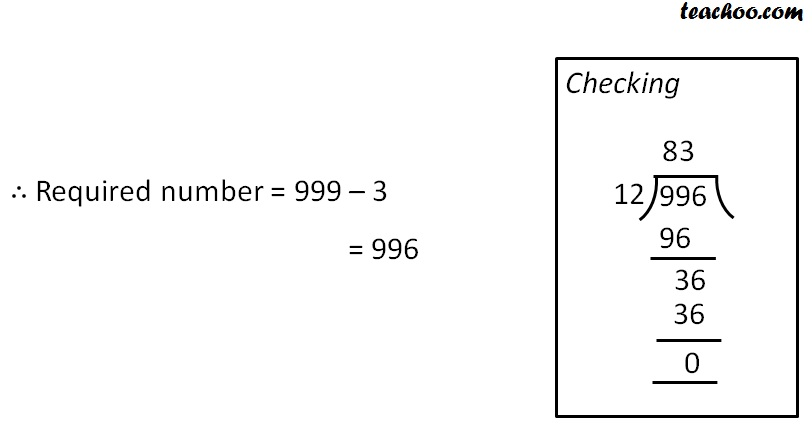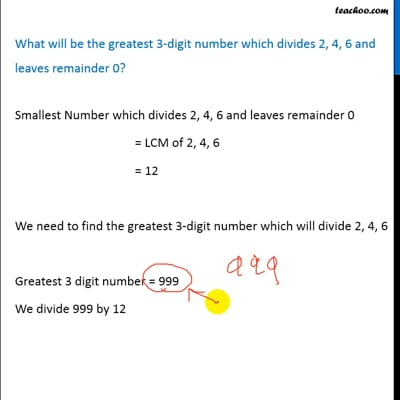Statement questions on LCM

Chapter 3 Class 6 Playing with Numbers
Concept wise

Smallest Number which divides 2, 4, 6 and leaves remainder 0

= LCM of 2, 4, 6

= 12

We need to find the greatest 3-digit number which will divide 2, 4, 6

Greatest 3 digit number = 999

We divide 999 by 12So remainder is 3

But we need to find a number where remainder is 0.So, 996 is the greatest 3 digit numberThis video is only available for Teachoo black users

### Transcript

What will be the greatest 3-digit number which divides 2, 4, 6 and leaves remainder 0? Smallest Number which divides 2, 4, 6 and leaves remainder 0 = LCM of 2, 4, 6 = 12 We need to find the greatest 3-digit number which will divide 2, 4, 6 Greatest 3 digit number = 999 We divide 999 by 12 So remainder is 3 But we need to find a number where remainder is 0. Required number = 999 3 = 996 So, 996 is the greatest 3 digit number